# Putting the symbolic linguistics series on indefinite hiatus

tl;dr: The scoping on this series was bad. It should be a book. I released the code under an MIT license on Github as treebender. There's a tutorial there. I'm planning posting some non-series intermediate Rust posts next. Thanks for reading the blog, I appreciate it.

As readers of this blog may be aware of, I have been writing a series about implementing an earley parser for linguistic utterances. This parser is being used for my in-development game Themengi, a game about learning an alien language, but I also was writing the series in part to advocate for a symbolic framework of parsing as better, in certain situations, than the dominant statistical / neural approach.

I published the first post — Why?, and some theory, in April. Astute readers may notice that it is now October, coincidentally exactly 6 months after I published that first post. Considering that my original goal was to write 5 posts, even if the second post was done and ready to publish that is not an encouraging schedule.

I could list a few reasons why my writing pace hasn't kept up with my goals — I graduated in August and started a new job shortly after (strive.co — we're hiring! Email me!), I also got married over the summer, the... everything happening in the world, etc. — and that's all true. However, the biggest problem has actually been my very inaccurate scoping of the project from the start. By a naive word count, the first post in this series was roughly 3100 words. My draft of the second post is currently sitting at almost 10,000 words, and is only halfway done. It barely has any code snippets, even though the series is advertised as implementing a parser, not just describing one in handwave-y detail.

So what happened? I think the scope for the series was wrong from the start. I didn't plan out the outline of the series past a very high level. I wrote basically an entire library, started using it, thought "oh, a blog series will be a nice way to introduce people to this!", sketched out a basic 5-part outline, and started writing. This made sense at the time because I expected the series to total about 10,000 words. When the first post came out at 3100 and I realized it didn't even have any code... I started worrying, but plowed ahead. 10k words into part 2, and I know for sure the scope on this is all wrong.

The definitive text on HDPSG, the framework that inspired this project, is "Syntactic Theory: A Formal Introduction". It is a textbook. It is 550 pages long. Granted, that includes exercises and some linguistic divergences I didn't plan to cover, but my scope also included some technical details that textbook glosses over. Looking over what I've written so far, I think that with what I've planned, there's enough material for at least a small book.1

I did say hiatus in the title though, and I do mean that. I'd like to come back to this series one day. However, I did also say indefinite, and that's also true. Might be never, or it might actually be a book, in the distant future where I've won the lottery and can afford to sit around writing technical books that only 3 people will ever read.

##What's happening to the code and to Themengi?

I wrote all the code for the library and had it almost working before I even finished writing the first post. All it took was a little cleaning and some documentation to get it to a publishable state, so it would have been pretty silly to not bring it over the finish line. So I took a couple days, wrote a short tutorial, and have published the library to Github as treebender. If you're interested in the project, I'd highly suggest going over to that repository and checking it out. The code is fairly readable, and the tutorial is pretty good if I can say so myself, so it should be a good way to jump in.

Likewise, Themengi (which has its own scoping issues and is taking forever) isn't going anywhere, and `treebender` will still have a place there.

##What's next for this blog?

Don't worry! I'm not giving up on the blog. If anything, clearing the air about this series should make it much easier for me to publish new posts, now that I don't have to feel guilty about not working on this series instead. My plans are to start working on some posts about intermediate Rust. I feel like there's no shortage of posts about "the Rust borrow checker changed the way I think!", and that's fine, but once you get past week 2 of Rust and the borrow checker mostly makes sense, I feel there's somewhat of a lack of content for the next rungs of that ladder beyond Programming Rust, which is both a bit outdated and a bit of a doorstop (in the most loving way). So keep an eye out for that.

If that interests you, and you haven't yet, there's a few ways you can follow me to get updates on new posts. You can subscribe to the RSS feed, follow me on Twitter, or subscribe to the mailing list.

##Oh, and one more thing...

Even though I'm not going to publish a part 2 anytime soon, I did want to at least show some of the visualizations I made, since I spent a lot of time on them, and I think they might be useful as inspiration for someone else who wants to illustrate the Earley algorithm. So for your viewing pleasure, free from commentary:

```chart := [ // 👀
(0, S -> • N IV)
(0, S -> • N TV N)
(0, S -> • N CV Comp S)
]
chart := []
chart := []
chart := []
chart := []
chart := []
chart := []
``````chart := [ // 👀
(0, S -> • N IV)
(0, S -> • N TV N)
(0, S -> • N CV Comp S)
(0, N -> • mary)
(0, N -> • sue)
(0, N -> • takeshi)
(0, N -> • robert)
]
chart := []
chart := []
chart := []
chart := []
chart := []
chart := []
```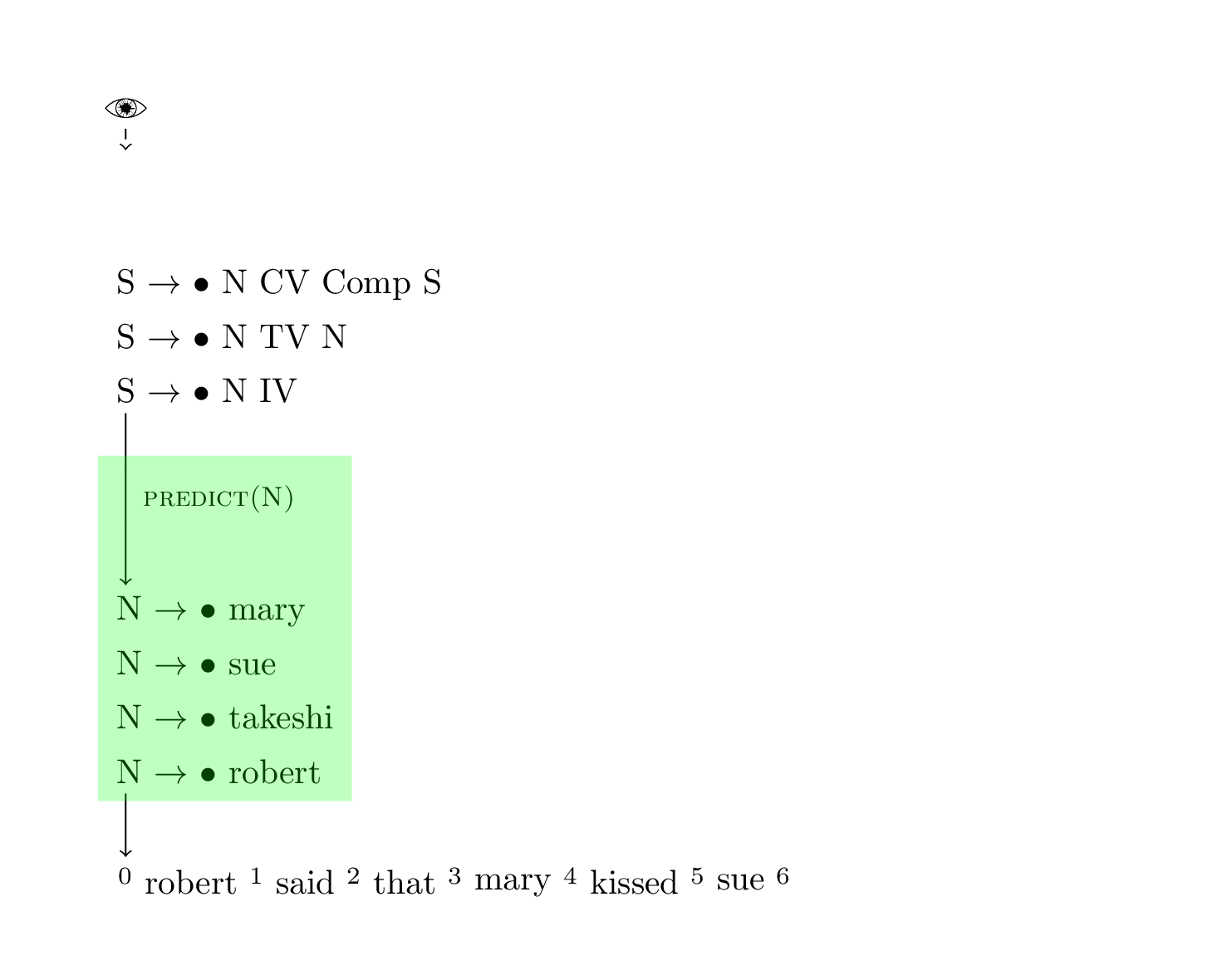```chart := [ // 👀
(0, S -> • N IV)
(0, S -> • N TV N)
(0, S -> • N CV Comp S)
(0, N -> • mary)
(0, N -> • sue)
(0, N -> • takeshi)
]
chart := [
(0, N -> robert •)
]
chart := []
chart := []
chart := []
chart := []
chart := []
```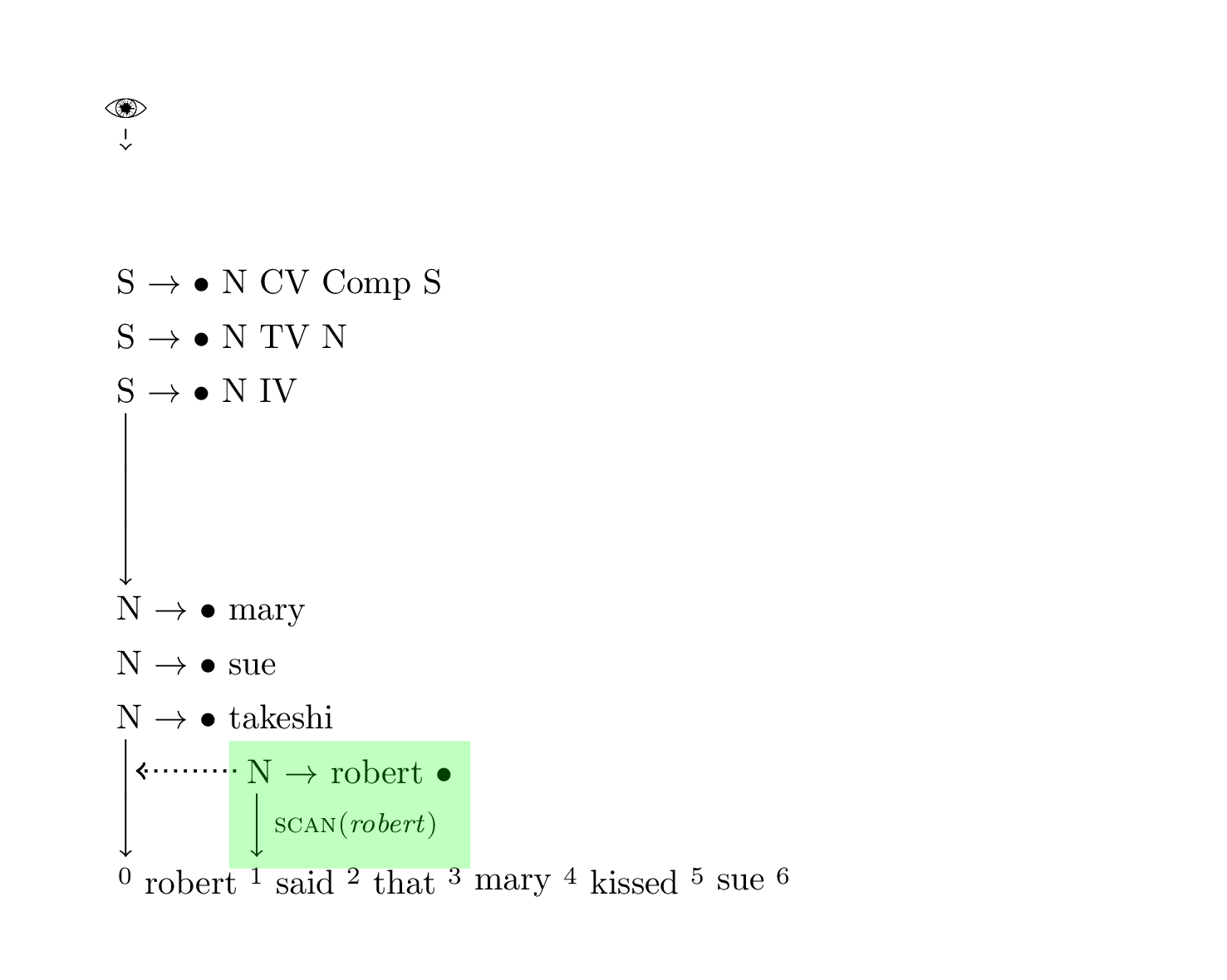```chart := [
(0, S -> • N IV)
(0, S -> • N TV N)
(0, S -> • N CV Comp S)
(0, N -> • mary)
(0, N -> • sue)
(0, N -> • takeshi)
]
chart := [ // 👀
(0, N -> robert •)
]
chart := []
chart := []
chart := []
chart := []
chart := []
``````chart := [
(0, N -> • mary)
(0, N -> • sue)
(0, N -> • takeshi)
]
chart := [ // 👀
(0, N -> robert ••)
(0, S -> N • IV)
(0, S -> N • TV N)
(0, S -> N • CV Comp S)
]
chart := []
chart := []
chart := []
chart := []
chart := []
```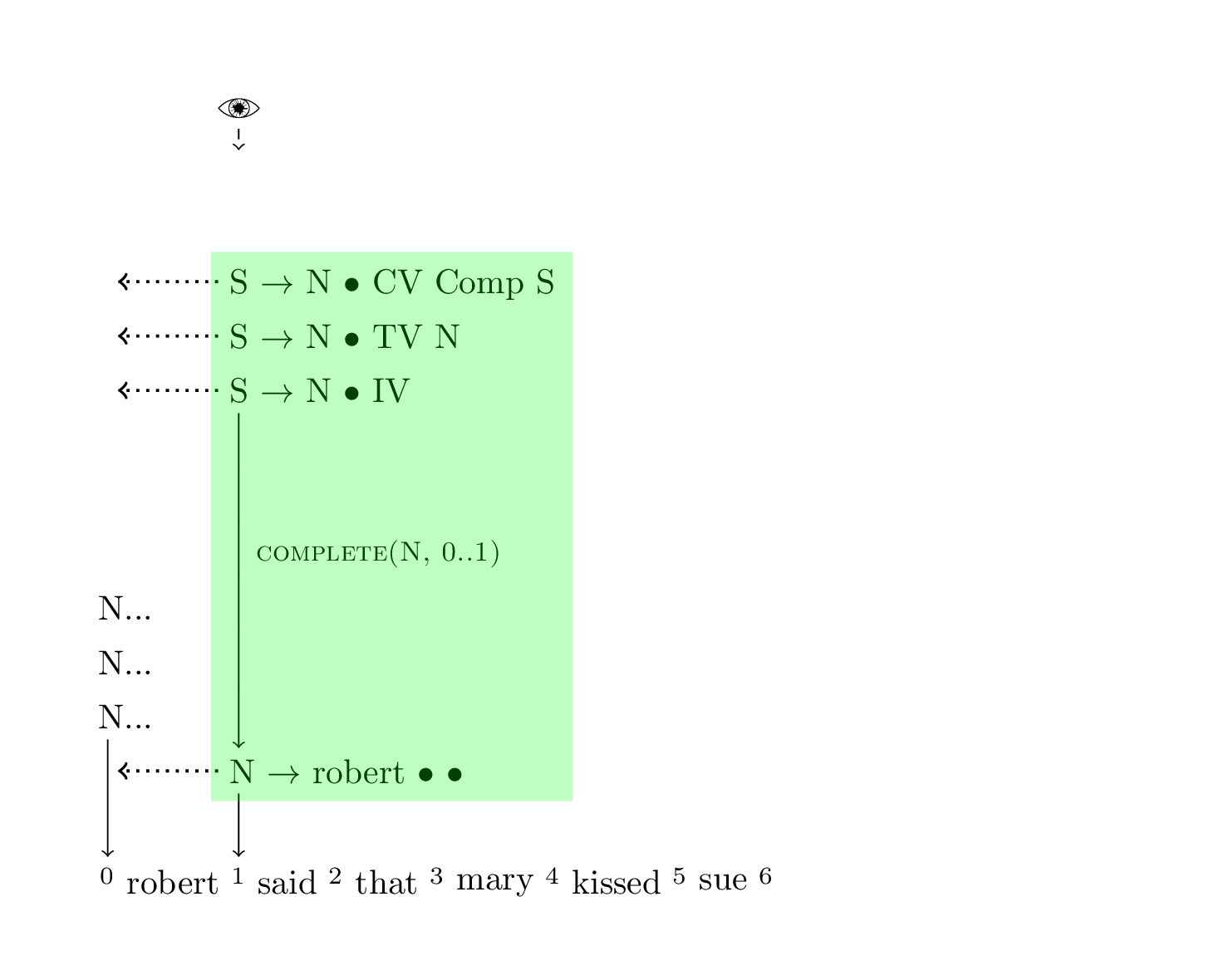```chart := [
(0, N -> • mary)
(0, N -> • sue)
(0, N -> • takeshi)
]
chart := [ // 👀
(0, N -> robert ••)
(0, S -> N • IV)
(0, S -> N • TV N)
(0, S -> N • CV Comp S)
]
chart := []
chart := []
chart := []
chart := []
chart := []
``````chart := [
(0, N -> • mary)
(0, N -> • sue)
(0, N -> • takeshi)
]
chart := [ // 👀
(0, N -> robert ••)
(0, S -> N • IV)
(0, S -> N • TV N)
(0, S -> N • CV Comp S)
(1, IV -> • fell)
(1, TV -> • kissed)
(1, CV -> • said)
(1, CV -> • thought)
]
chart := []
chart := []
chart := []
chart := []
chart := []
```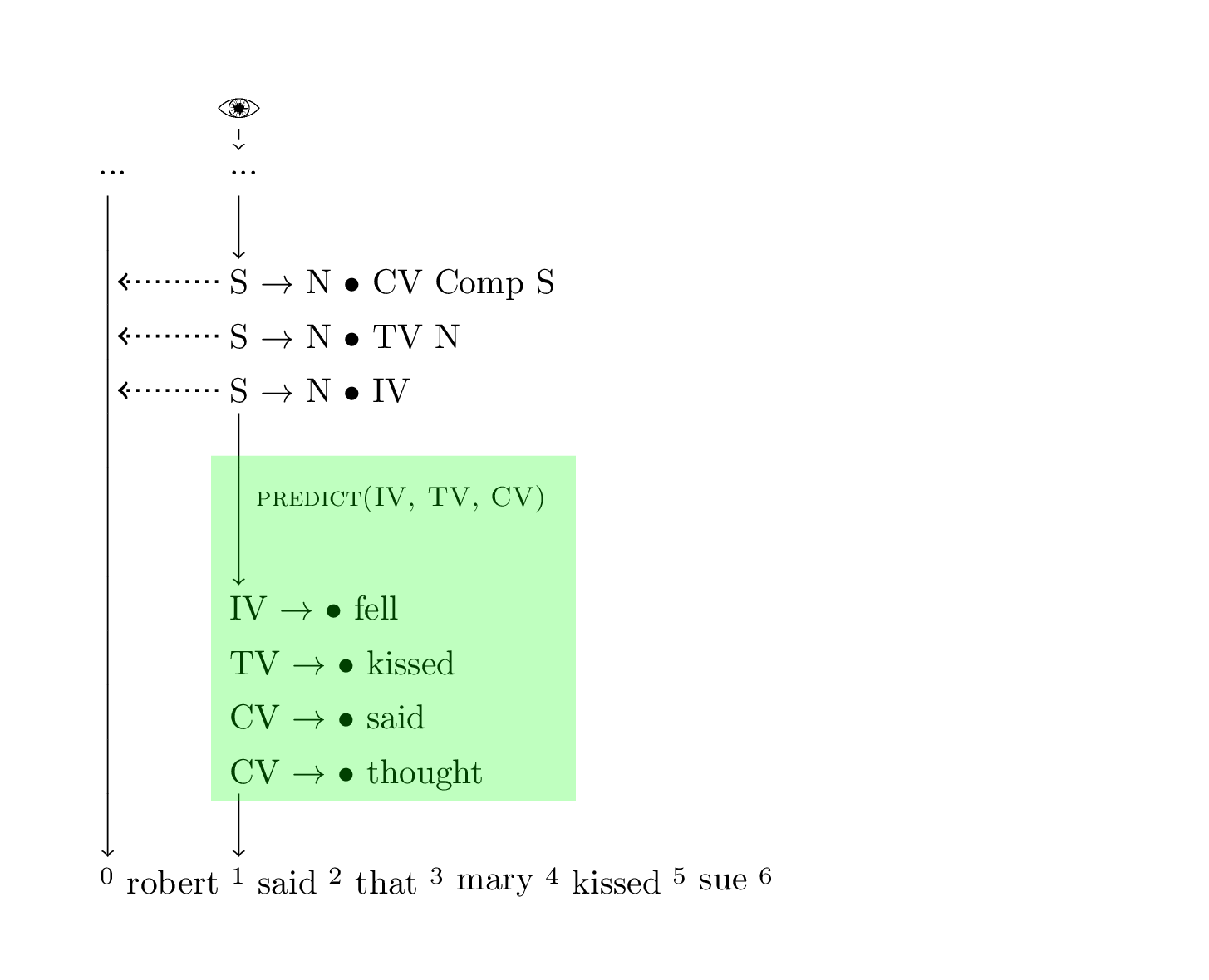```chart := [
(0, N -> • mary)
(0, N -> • sue)
(0, N -> • takeshi)
]
chart := [ // 👀
(0, N -> robert ••)
(0, S -> N • IV)
(0, S -> N • TV N)
(0, S -> N • CV Comp S)
(1, IV -> • fell)
(1, TV -> • kissed)
(1, CV -> • thought)
]
chart := [
(1, CV -> said •)
]
chart := []
chart := []
chart := []
chart := []
```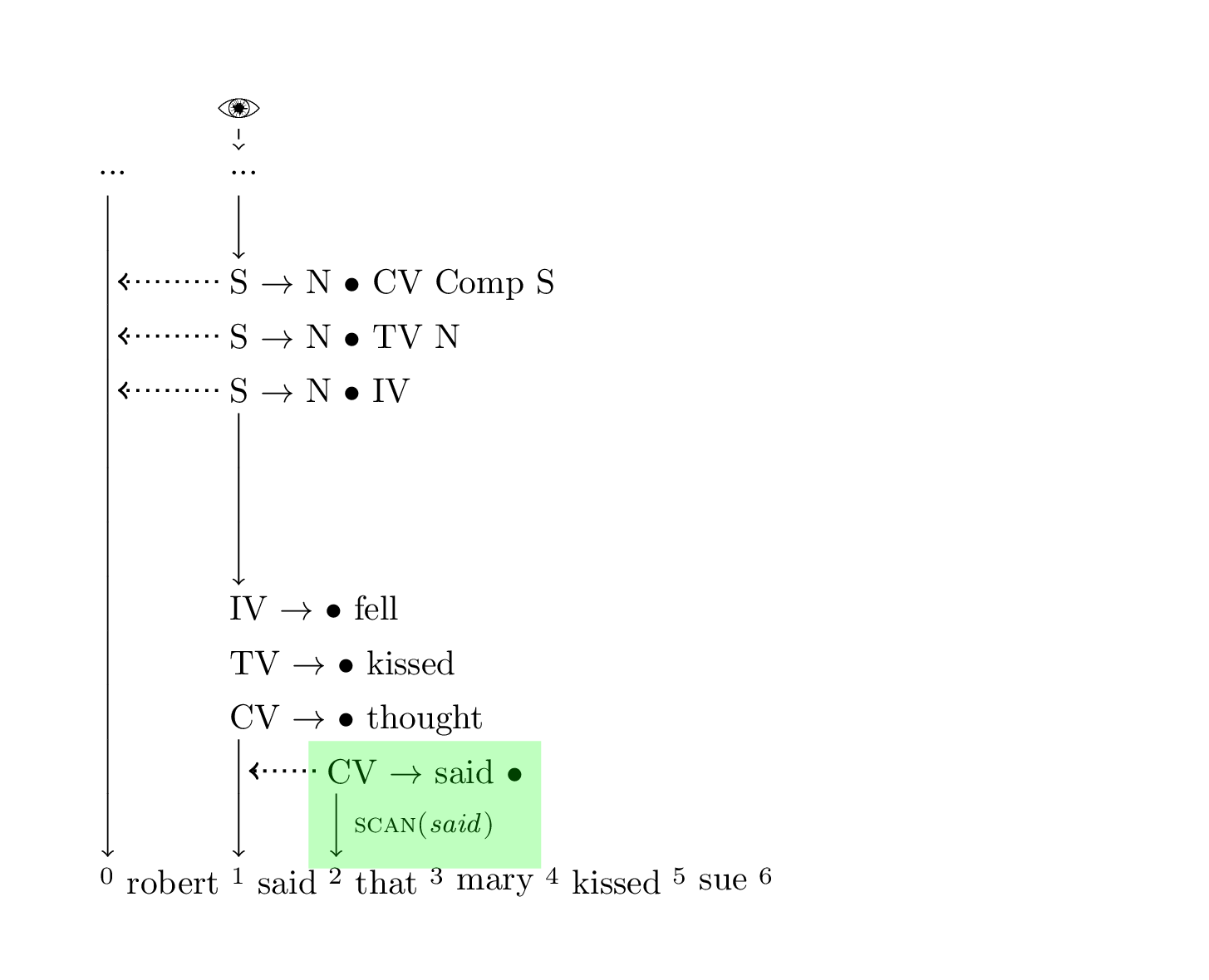```chart := [
(0, N -> • mary)
(0, N -> • sue)
(0, N -> • takeshi)
]
chart := [
(0, N -> robert ••)
(0, S -> N • IV)
(0, S -> N • TV N)
(0, S -> N • CV Comp S)
(1, IV -> • fell)
(1, TV -> • kissed)
(1, CV -> • thought)
]
chart := [ // 👀
(1, CV -> said •)
]
chart := []
chart := []
chart := []
chart := []
``````chart := [
(0, N -> • mary)
(0, N -> • sue)
(0, N -> • takeshi)
]
chart := [
(0, N -> robert ••)
(0, S -> N • IV)
(0, S -> N • TV N)
(1, IV -> • fell)
(1, TV -> • kissed)
(1, CV -> • thought)
]
chart := [ // 👀
(1, CV -> said ••)
(0, S -> N CV • Comp S)
]
chart := []
chart := []
chart := []
chart := []
```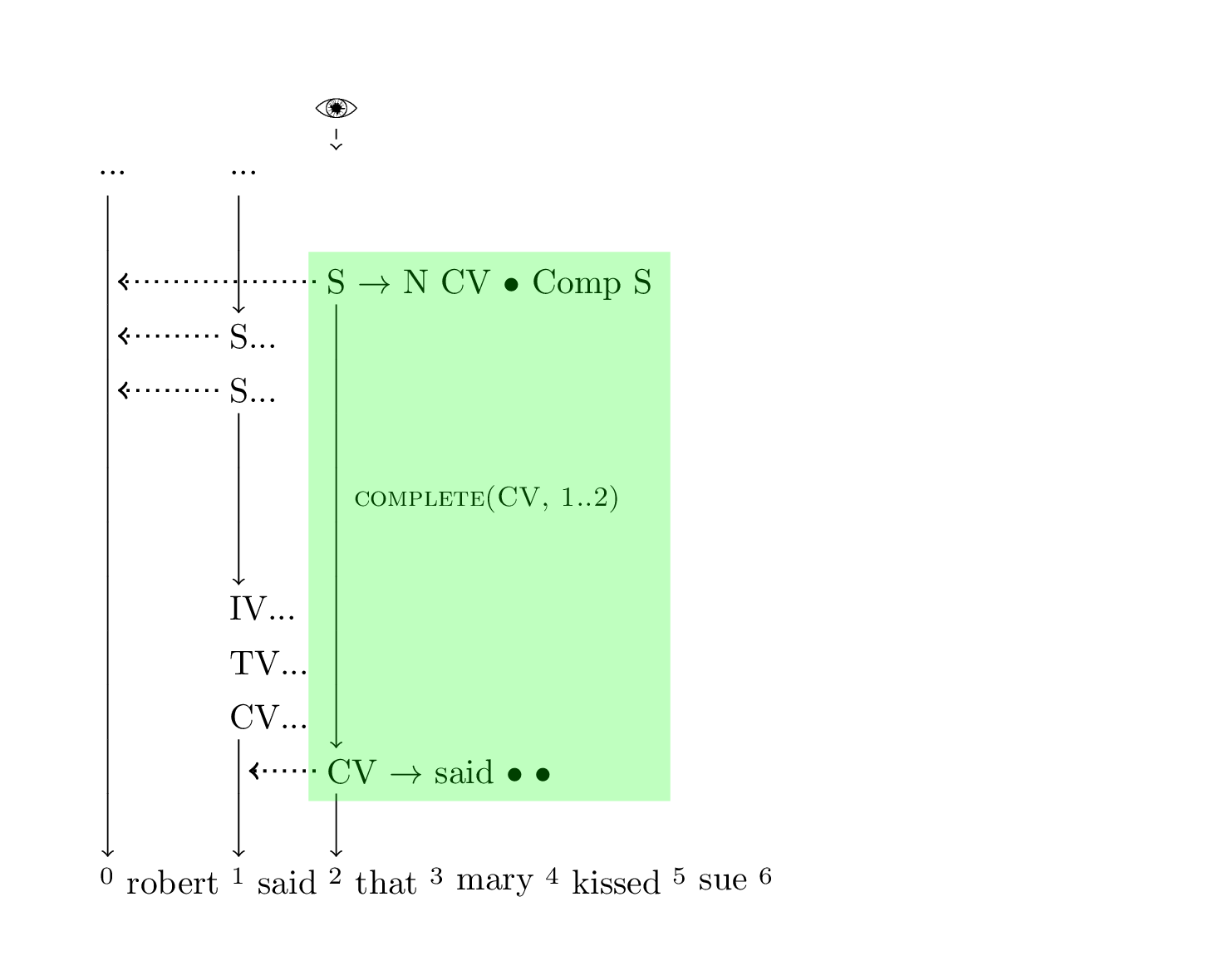```chart := [
(0, N -> • mary)
(0, N -> • sue)
(0, N -> • takeshi)
]
chart := [
(0, N -> robert ••)
(0, S -> N • IV)
(0, S -> N • TV N)
(1, IV -> • fell)
(1, TV -> • kissed)
(1, CV -> • thought)
]
chart := [ // 👀
(1, CV -> said ••)
(0, S -> N CV • Comp S)
]
chart := []
chart := []
chart := []
chart := []
``````chart := [
(0, N -> • mary)
(0, N -> • sue)
(0, N -> • takeshi)
]
chart := [
(0, N -> robert ••)
(0, S -> N • IV)
(0, S -> N • TV N)
(1, IV -> • fell)
(1, TV -> • kissed)
(1, CV -> • thought)
]
chart := [ // 👀
(1, CV -> said ••)
(0, S -> N CV • Comp S)
(2, Comp -> • that)
]
chart := []
chart := []
chart := []
chart := []
```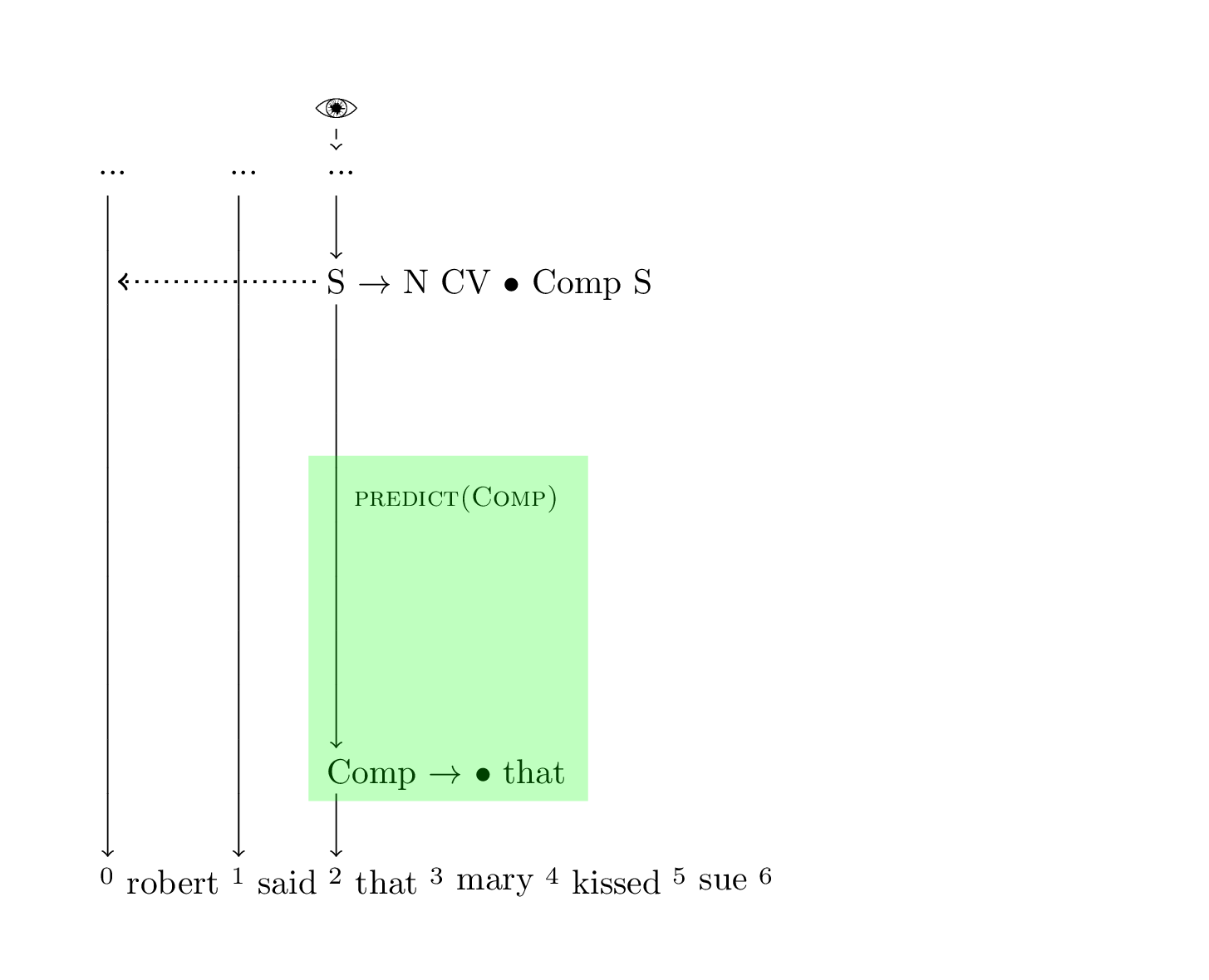```chart := [
(0, N -> • mary)
(0, N -> • sue)
(0, N -> • takeshi)
]
chart := [
(0, N -> robert ••)
(0, S -> N • IV)
(0, S -> N • TV N)
(1, IV -> • fell)
(1, TV -> • kissed)
(1, CV -> • thought)
]
chart := [ // 👀
(1, CV -> said ••)
(0, S -> N CV • Comp S)
]
chart := [
(2, Comp -> that •)
]
chart := []
chart := []
chart := []
```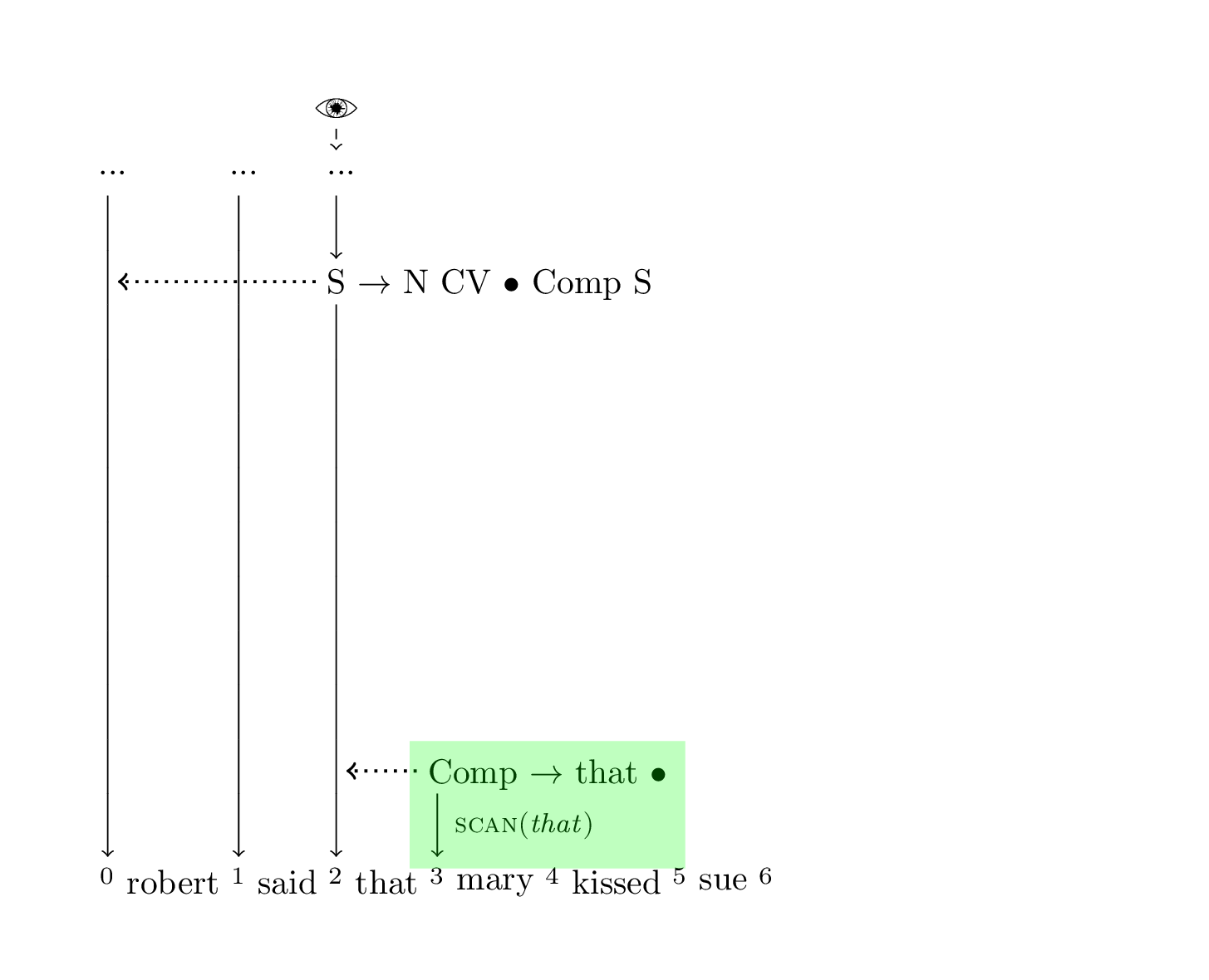```chart := [
(0, N -> • mary)
(0, N -> • sue)
(0, N -> • takeshi)
]
chart := [
(0, N -> robert ••)
(0, S -> N • IV)
(0, S -> N • TV N)
(1, IV -> • fell)
(1, TV -> • kissed)
(1, CV -> • thought)
]
chart := [
(1, CV -> said ••)
(0, S -> N CV • Comp S)
]
chart := [ // 👀
(2, Comp -> that •)
]
chart := []
chart := []
chart := []
``````chart := [
(0, N -> • mary)
(0, N -> • sue)
(0, N -> • takeshi)
]
chart := [
(0, N -> robert ••)
(0, S -> N • IV)
(0, S -> N • TV N)
(1, IV -> • fell)
(1, TV -> • kissed)
(1, CV -> • thought)
]
chart := [
(1, CV -> said ••)
]
chart := [ // 👀
(2, Comp -> that ••)
(0, S -> N CV Comp • S)
]
chart := []
chart := []
chart := []
```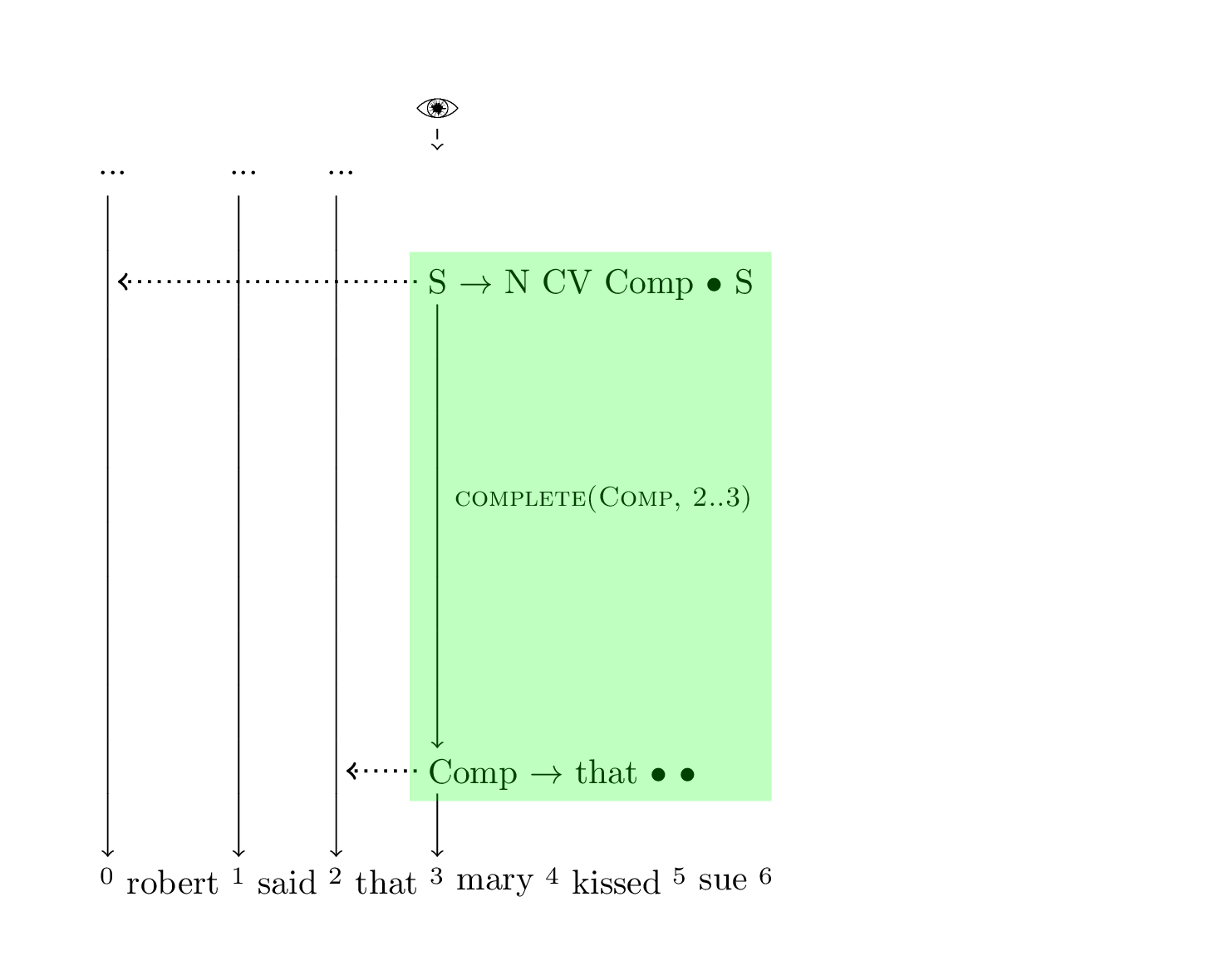```chart := [
(0, N -> • mary)
(0, N -> • sue)
(0, N -> • takeshi)
]
chart := [
(0, N -> robert ••)
(0, S -> N • IV)
(0, S -> N • TV N)
(1, IV -> • fell)
(1, TV -> • kissed)
(1, CV -> • thought)
]
chart := [
(1, CV -> said ••)
]
chart := [ // 👀
(2, Comp -> that ••)
(0, S -> N CV Comp • S)
]
chart := []
chart := []
chart := []
``````chart := [
(0, N -> • mary)
(0, N -> • sue)
(0, N -> • takeshi)
]
chart := [
(0, N -> robert ••)
(0, S -> N • IV)
(0, S -> N • TV N)
(1, IV -> • fell)
(1, TV -> • kissed)
(1, CV -> • thought)
]
chart := [
(1, CV -> said ••)
]
chart := [ // 👀
(2, Comp -> that ••)
(0, S -> N CV Comp • S)
(3, S -> • N IV)
(3, S -> • N CV Comp S)
(3, S -> • N TV N)
]
chart := []
chart := []
chart := []
```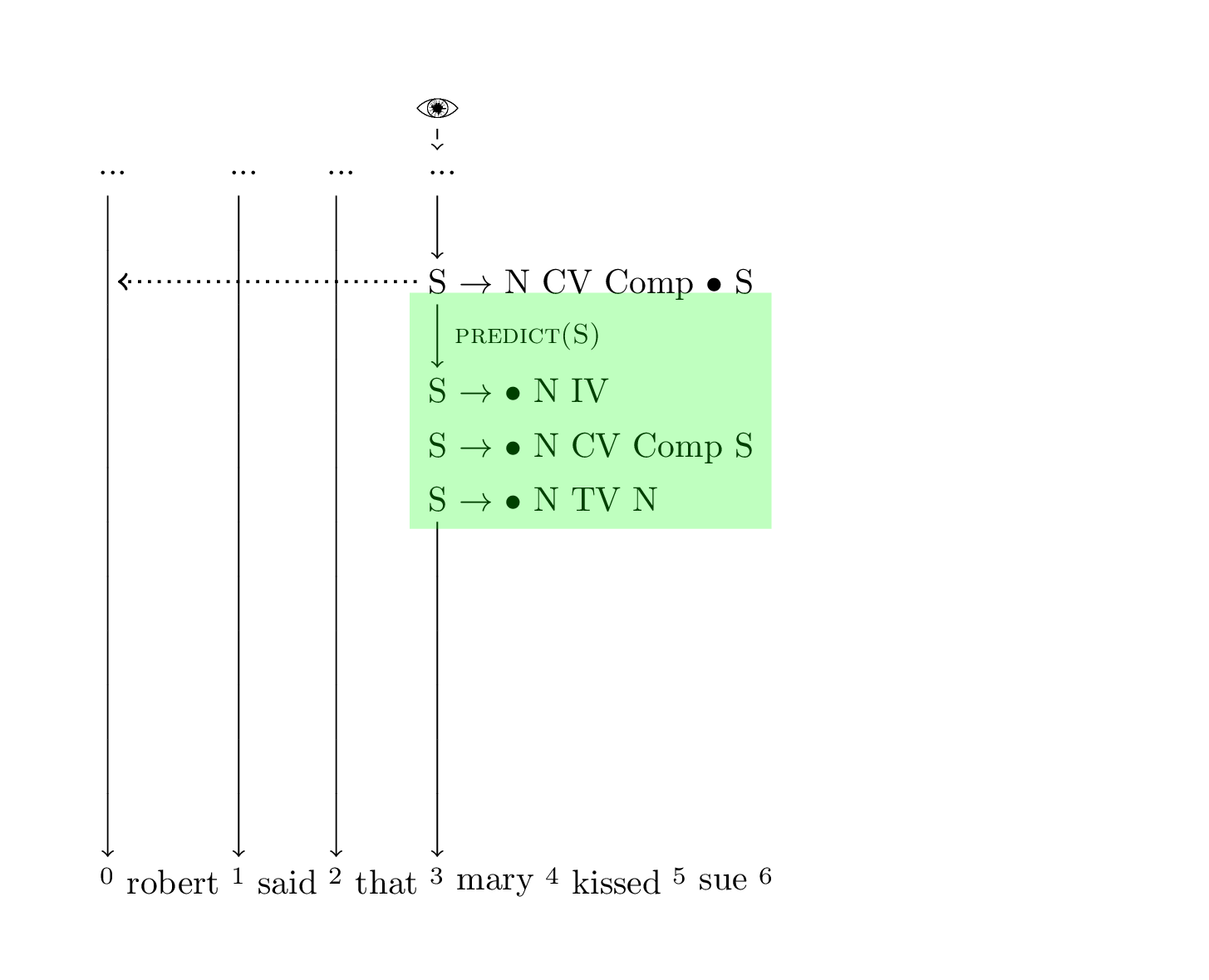```chart := [
(0, N -> • mary)
(0, N -> • sue)
(0, N -> • takeshi)
]
chart := [
(0, N -> robert ••)
(0, S -> N • IV)
(0, S -> N • TV N)
(1, IV -> • fell)
(1, TV -> • kissed)
(1, CV -> • thought)
]
chart := [
(1, CV -> said ••)
]
chart := [ // 👀
(2, Comp -> that ••)
(0, S -> N CV Comp • S)
(3, S -> • N IV)
(3, S -> • N CV Comp S)
(3, S -> • N TV N)
(3, N -> • sue)
(3, N -> • robert)
(3, N -> • takeshi)
(3, N -> • mary)
]
chart := []
chart := []
chart := []
```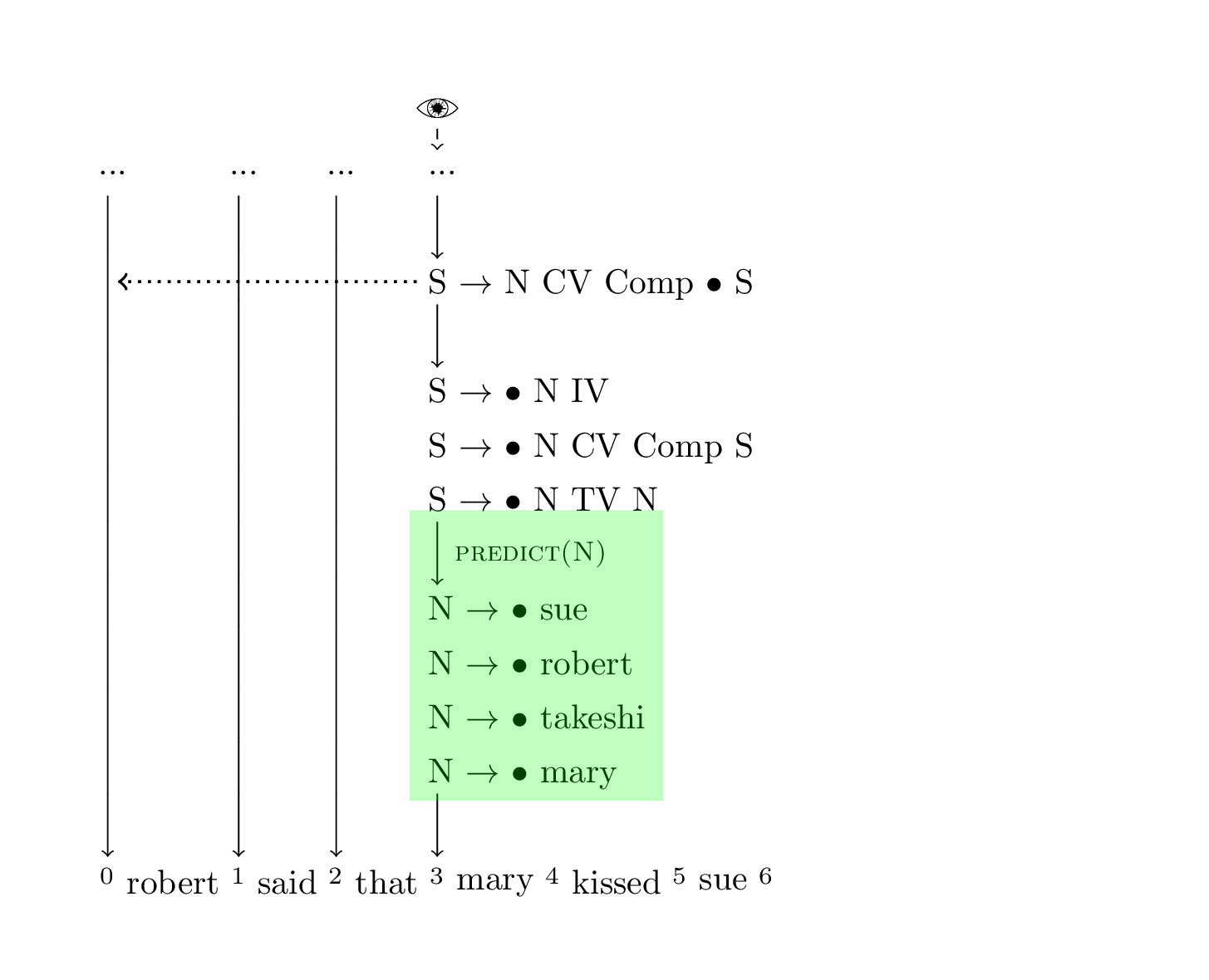```chart := [
(0, N -> • mary)
(0, N -> • sue)
(0, N -> • takeshi)
]
chart := [
(0, N -> robert ••)
(0, S -> N • IV)
(0, S -> N • TV N)
(1, IV -> • fell)
(1, TV -> • kissed)
(1, CV -> • thought)
]
chart := [
(1, CV -> said ••)
]
chart := [ // 👀
(2, Comp -> that ••)
(0, S -> N CV Comp • S)
(3, S -> • N IV)
(3, S -> • N CV Comp S)
(3, S -> • N TV N)
(3, N -> • sue)
(3, N -> • robert)
(3, N -> • takeshi)
]
chart := [
(3, N -> mary •)
]
chart := []
chart := []
```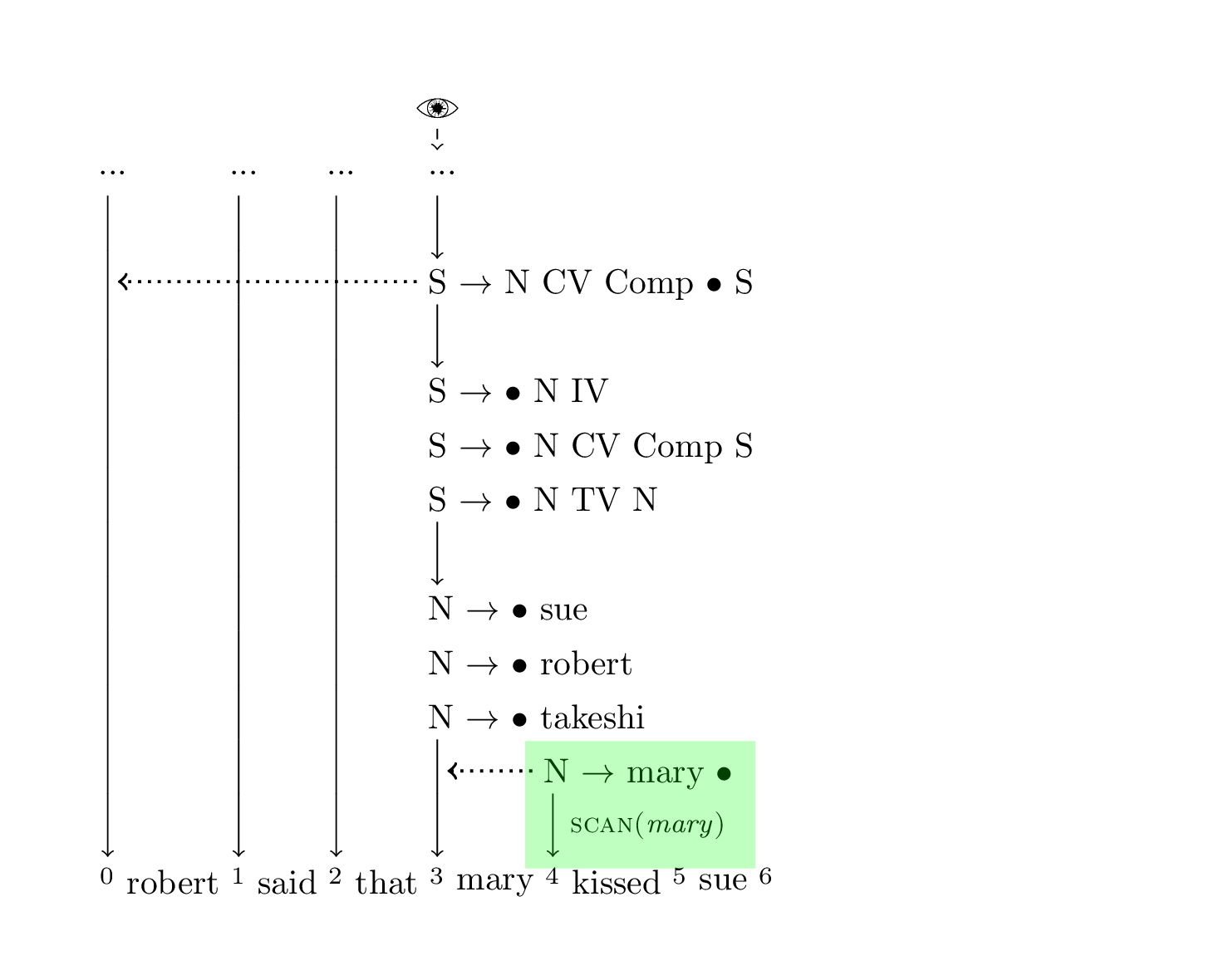```chart := [
(0, N -> • mary)
(0, N -> • sue)
(0, N -> • takeshi)
]
chart := [
(0, N -> robert ••)
(0, S -> N • IV)
(0, S -> N • TV N)
(1, IV -> • fell)
(1, TV -> • kissed)
(1, CV -> • thought)
]
chart := [
(1, CV -> said ••)
]
chart := [
(2, Comp -> that ••)
(0, S -> N CV Comp • S)
(3, S -> • N IV)
(3, S -> • N CV Comp S)
(3, S -> • N TV N)
(3, N -> • sue)
(3, N -> • robert)
(3, N -> • takeshi)
]
chart := [ // 👀
(3, N -> mary •)
]
chart := []
chart := []
``````chart := [
(0, N -> • mary)
(0, N -> • sue)
(0, N -> • takeshi)
]
chart := [
(0, N -> robert ••)
(0, S -> N • IV)
(0, S -> N • TV N)
(1, IV -> • fell)
(1, TV -> • kissed)
(1, CV -> • thought)
]
chart := [
(1, CV -> said ••)
]
chart := [
(2, Comp -> that ••)
(0, S -> N CV Comp • S)
(3, N -> • sue)
(3, N -> • robert)
(3, N -> • takeshi)
]
chart := [ // 👀
(3, N -> mary ••)
(3, S -> N • IV)
(3, S -> N • CV Comp S)
(3, S -> N • TV N)
]
chart := []
chart := []
```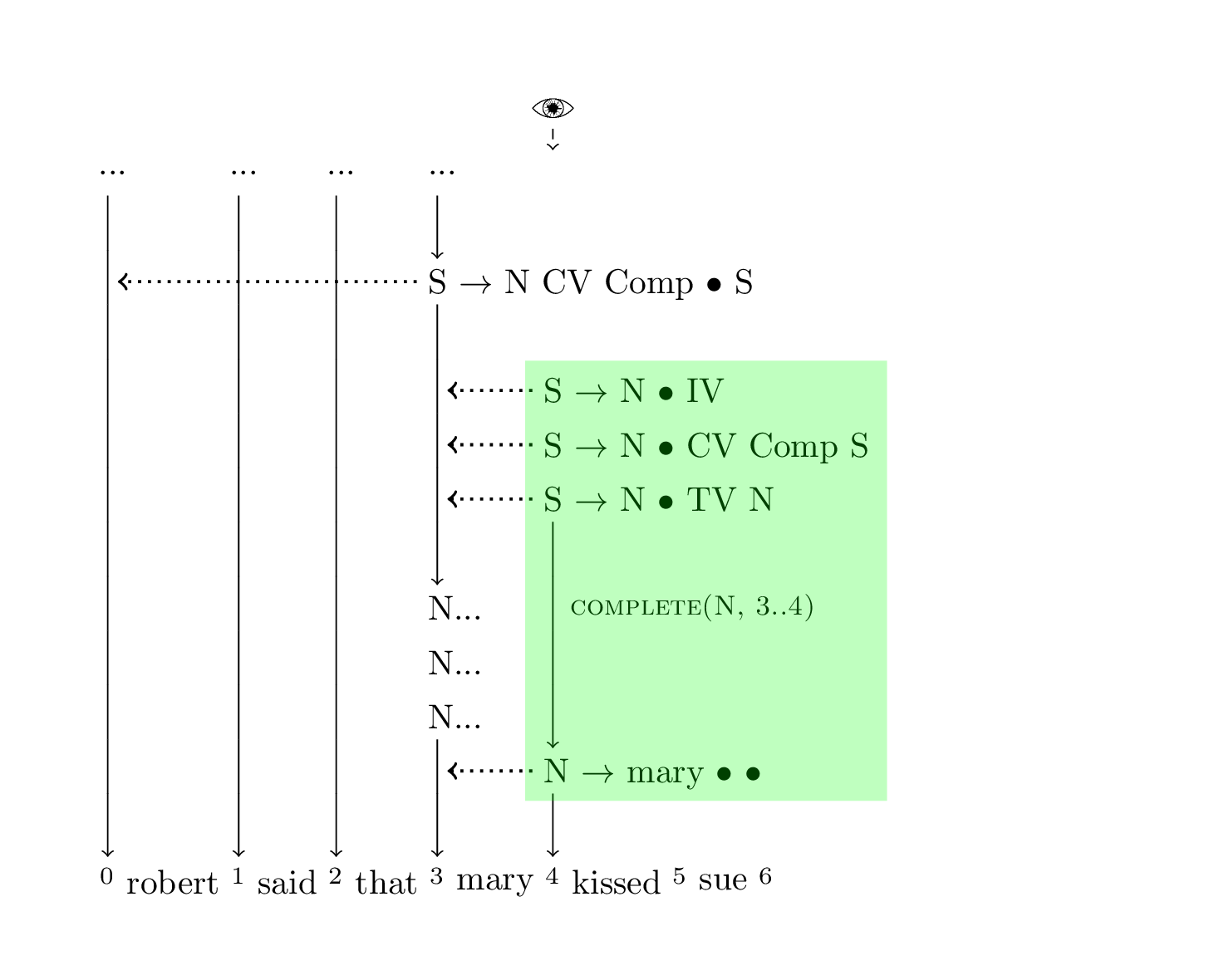```chart := [
(0, N -> • mary)
(0, N -> • sue)
(0, N -> • takeshi)
]
chart := [
(0, N -> robert ••)
(0, S -> N • IV)
(0, S -> N • TV N)
(1, IV -> • fell)
(1, TV -> • kissed)
(1, CV -> • thought)
]
chart := [
(1, CV -> said ••)
]
chart := [
(2, Comp -> that ••)
(0, S -> N CV Comp • S)
(3, N -> • sue)
(3, N -> • robert)
(3, N -> • takeshi)
]
chart := [ // 👀
(3, N -> mary ••)
(3, S -> N • IV)
(3, S -> N • CV Comp S)
(3, S -> N • TV N)
]
chart := []
chart := []
``````chart := [
(0, N -> • mary)
(0, N -> • sue)
(0, N -> • takeshi)
]
chart := [
(0, N -> robert ••)
(0, S -> N • IV)
(0, S -> N • TV N)
(1, IV -> • fell)
(1, TV -> • kissed)
(1, CV -> • thought)
]
chart := [
(1, CV -> said ••)
]
chart := [
(2, Comp -> that ••)
(0, S -> N CV Comp • S)
(3, N -> • sue)
(3, N -> • robert)
(3, N -> • takeshi)
]
chart := [ // 👀
(3, N -> mary ••)
(3, S -> N • IV)
(3, S -> N • CV Comp S)
(3, S -> N • TV N)
(4, IV -> • fell)
(4, CV -> • said)
(4, CV -> • thought)
(4, TV -> • kissed)
]
chart := []
chart := []
```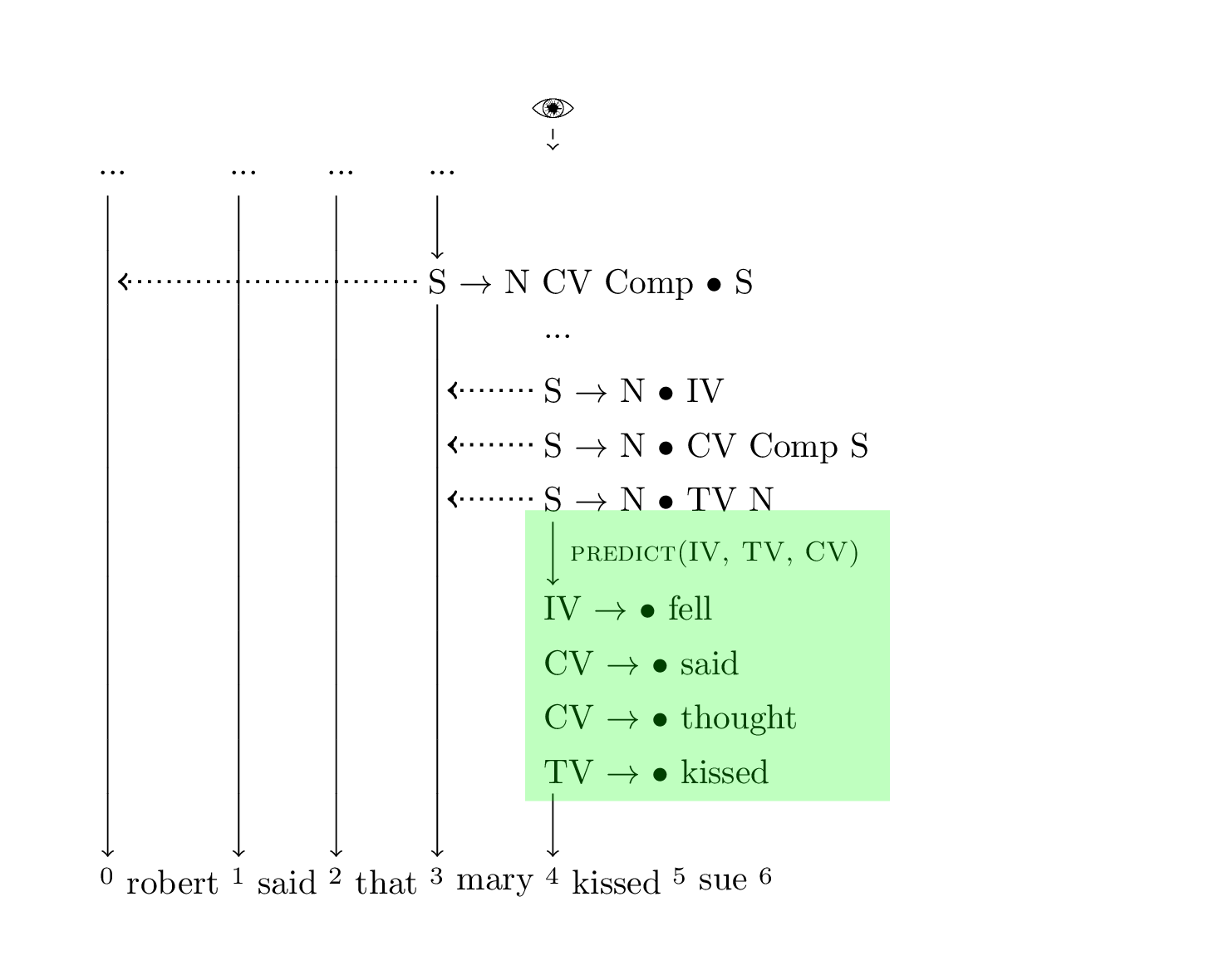```chart := [
(0, N -> • mary)
(0, N -> • sue)
(0, N -> • takeshi)
]
chart := [
(0, N -> robert ••)
(0, S -> N • IV)
(0, S -> N • TV N)
(1, IV -> • fell)
(1, TV -> • kissed)
(1, CV -> • thought)
]
chart := [
(1, CV -> said ••)
]
chart := [
(2, Comp -> that ••)
(0, S -> N CV Comp • S)
(3, N -> • sue)
(3, N -> • robert)
(3, N -> • takeshi)
]
chart := [ // 👀
(3, N -> mary ••)
(3, S -> N • IV)
(3, S -> N • CV Comp S)
(3, S -> N • TV N)
(4, IV -> • fell)
(4, CV -> • said)
(4, CV -> • thought)
]
chart := [
(4, TV -> kissed •)
]
chart := []
```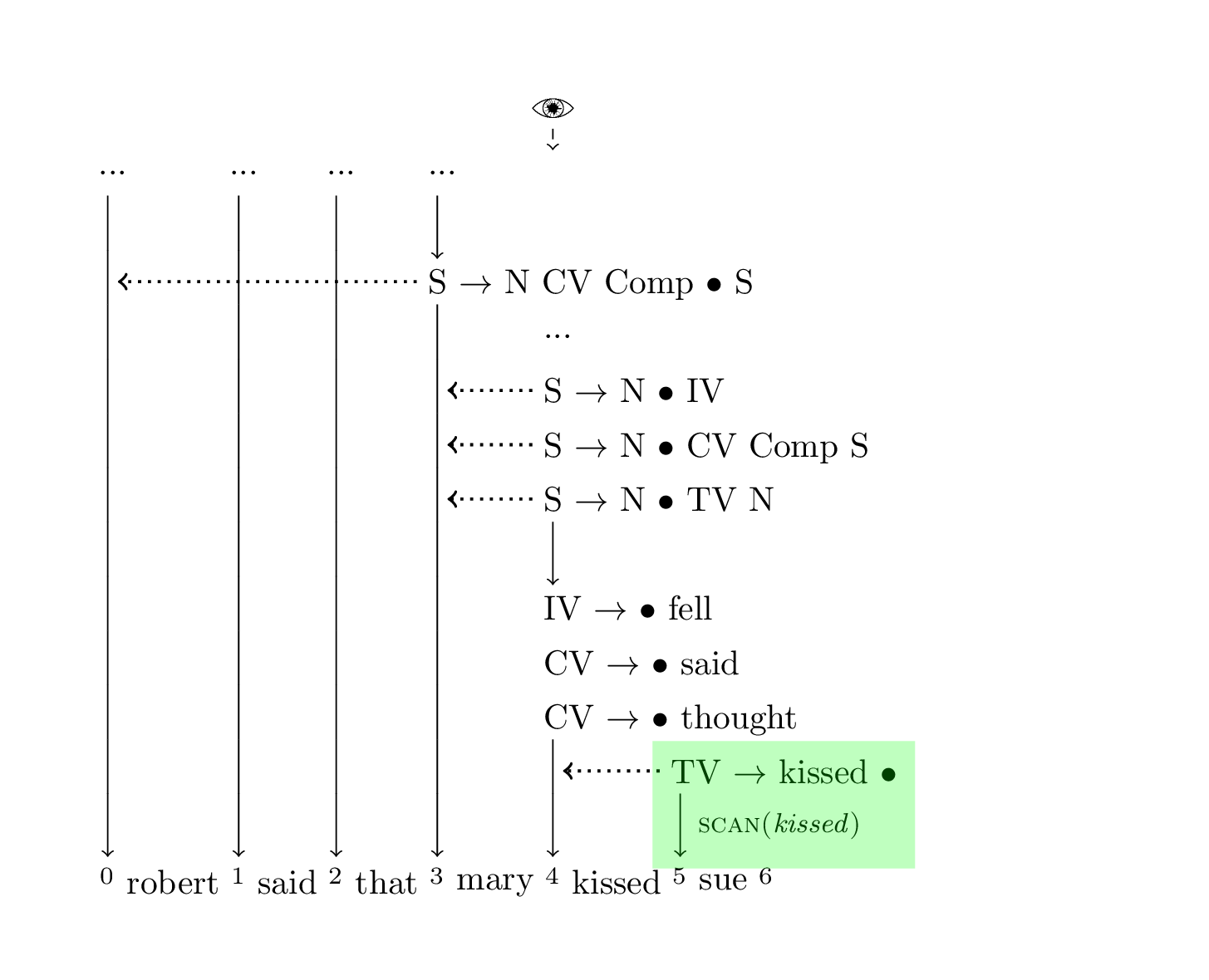```chart := [
(0, N -> • mary)
(0, N -> • sue)
(0, N -> • takeshi)
]
chart := [
(0, N -> robert ••)
(0, S -> N • IV)
(0, S -> N • TV N)
(1, IV -> • fell)
(1, TV -> • kissed)
(1, CV -> • thought)
]
chart := [
(1, CV -> said ••)
]
chart := [
(2, Comp -> that ••)
(0, S -> N CV Comp • S)
(3, N -> • sue)
(3, N -> • robert)
(3, N -> • takeshi)
]
chart := [
(3, N -> mary ••)
(3, S -> N • IV)
(3, S -> N • CV Comp S)
(3, S -> N • TV N)
(4, IV -> • fell)
(4, CV -> • said)
(4, CV -> • thought)
]
chart := [ // 👀
(4, TV -> kissed •)
]
chart := []
``````chart := [
(0, N -> • mary)
(0, N -> • sue)
(0, N -> • takeshi)
]
chart := [
(0, N -> robert ••)
(0, S -> N • IV)
(0, S -> N • TV N)
(1, IV -> • fell)
(1, TV -> • kissed)
(1, CV -> • thought)
]
chart := [
(1, CV -> said ••)
]
chart := [
(2, Comp -> that ••)
(0, S -> N CV Comp • S)
(3, N -> • sue)
(3, N -> • robert)
(3, N -> • takeshi)
]
chart := [
(3, N -> mary ••)
(3, S -> N • IV)
(3, S -> N • CV Comp S)
(4, IV -> • fell)
(4, CV -> • said)
(4, CV -> • thought)
]
chart := [ // 👀
(4, TV -> kissed ••)
(3, S -> N TV • N)
]
chart := []
```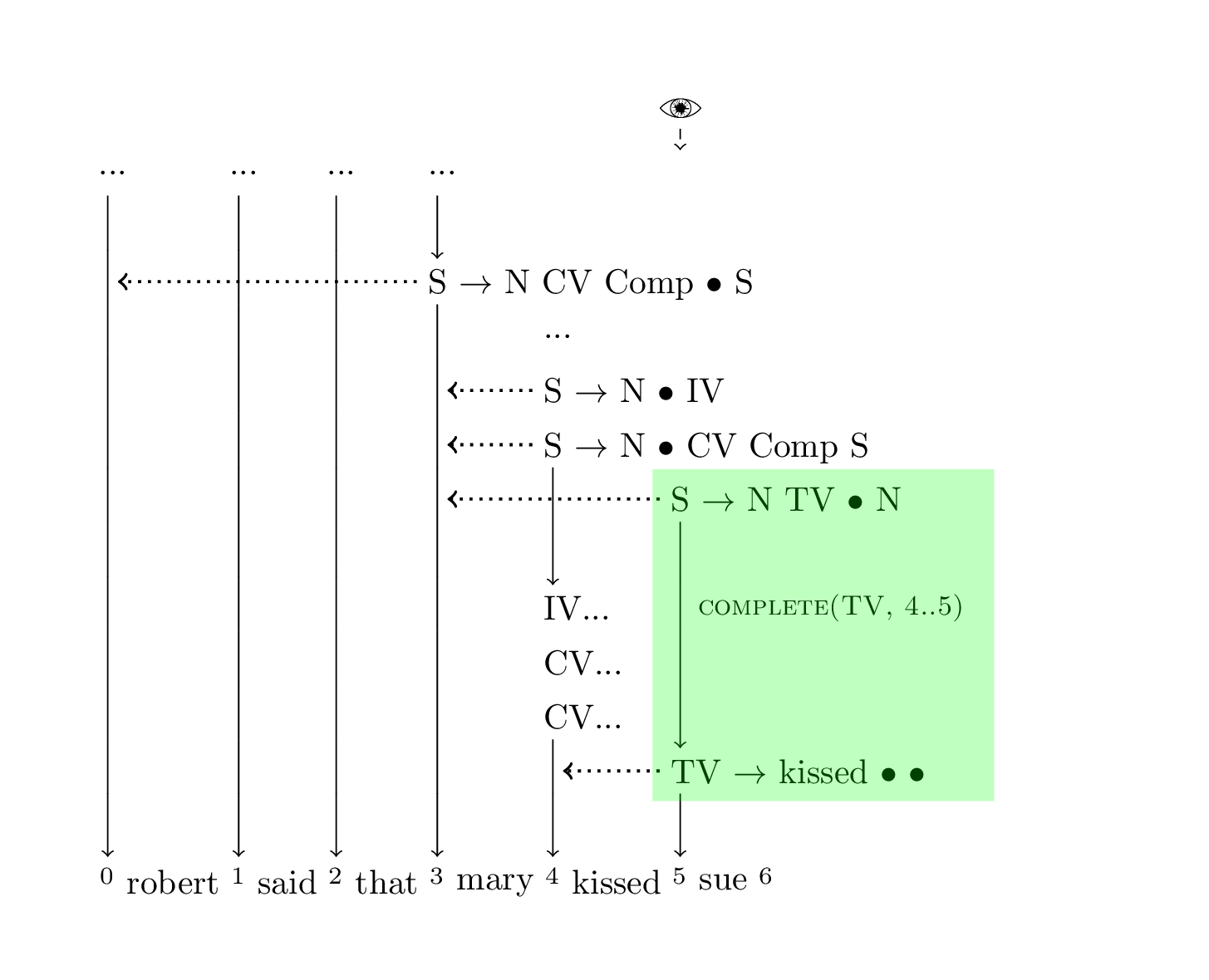```chart := [
(0, N -> • mary)
(0, N -> • sue)
(0, N -> • takeshi)
]
chart := [
(0, N -> robert ••)
(0, S -> N • IV)
(0, S -> N • TV N)
(1, IV -> • fell)
(1, TV -> • kissed)
(1, CV -> • thought)
]
chart := [
(1, CV -> said ••)
]
chart := [
(2, Comp -> that ••)
(0, S -> N CV Comp • S)
(3, N -> • sue)
(3, N -> • robert)
(3, N -> • takeshi)
]
chart := [
(3, N -> mary ••)
(3, S -> N • IV)
(3, S -> N • CV Comp S)
(4, IV -> • fell)
(4, CV -> • said)
(4, CV -> • thought)
]
chart := [ // 👀
(4, TV -> kissed ••)
(3, S -> N TV • N)
]
chart := []
``````chart := [
(0, N -> • mary)
(0, N -> • sue)
(0, N -> • takeshi)
]
chart := [
(0, N -> robert ••)
(0, S -> N • IV)
(0, S -> N • TV N)
(1, IV -> • fell)
(1, TV -> • kissed)
(1, CV -> • thought)
]
chart := [
(1, CV -> said ••)
]
chart := [
(2, Comp -> that ••)
(0, S -> N CV Comp • S)
(3, N -> • sue)
(3, N -> • robert)
(3, N -> • takeshi)
]
chart := [
(3, N -> mary ••)
(3, S -> N • IV)
(3, S -> N • CV Comp S)
(4, IV -> • fell)
(4, CV -> • said)
(4, CV -> • thought)
]
chart := [ // 👀
(4, TV -> kissed ••)
(3, S -> N TV • N)
(5, N -> • robert)
(5, N -> • takeshi)
(5, N -> • mary)
(5, N -> • sue)
]
chart := []
```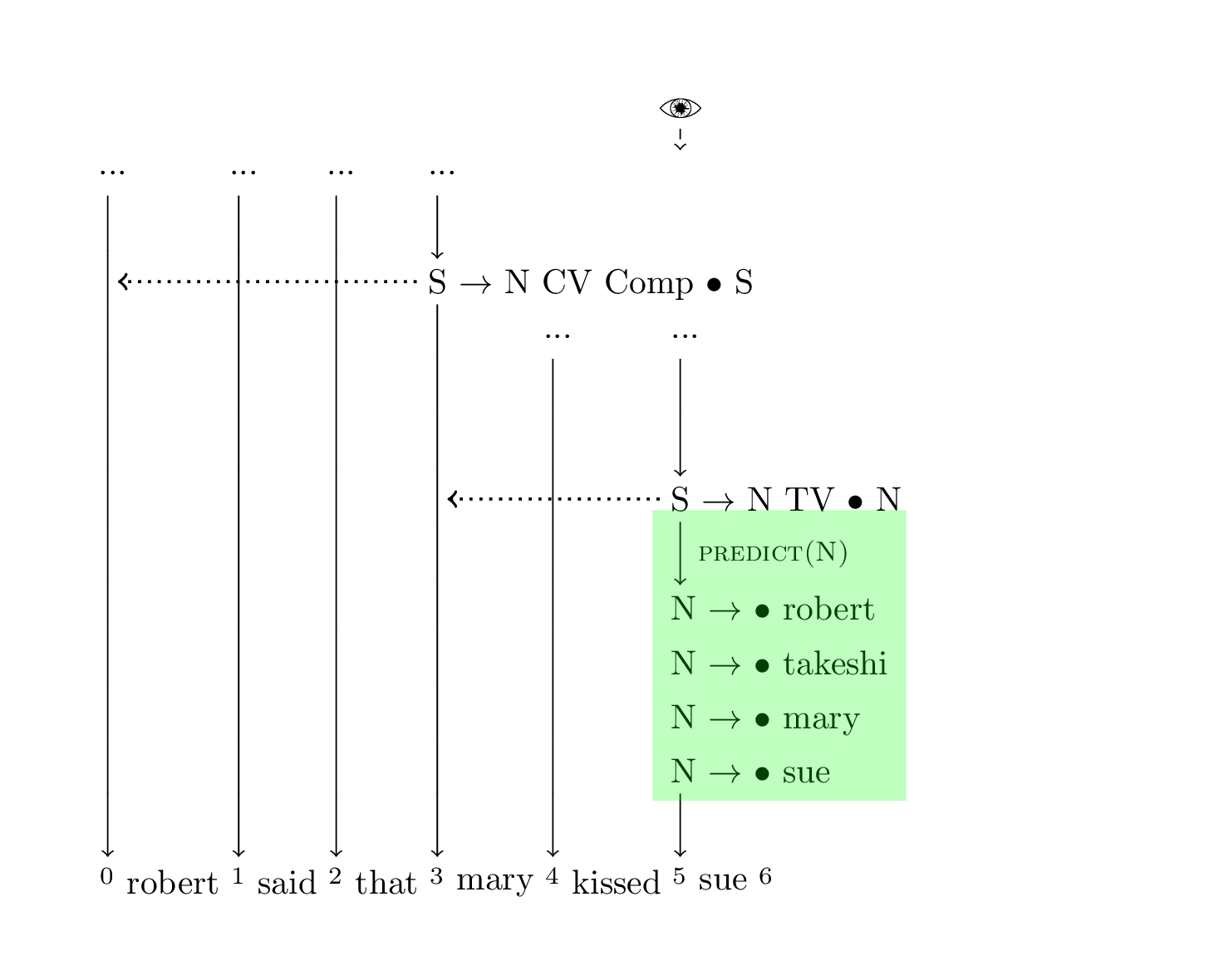```chart := [
(0, N -> • mary)
(0, N -> • sue)
(0, N -> • takeshi)
]
chart := [
(0, N -> robert ••)
(0, S -> N • IV)
(0, S -> N • TV N)
(1, IV -> • fell)
(1, TV -> • kissed)
(1, CV -> • thought)
]
chart := [
(1, CV -> said ••)
]
chart := [
(2, Comp -> that ••)
(0, S -> N CV Comp • S)
(3, N -> • sue)
(3, N -> • robert)
(3, N -> • takeshi)
]
chart := [
(3, N -> mary ••)
(3, S -> N • IV)
(3, S -> N • CV Comp S)
(4, IV -> • fell)
(4, CV -> • said)
(4, CV -> • thought)
]
chart := [ // 👀
(4, TV -> kissed ••)
(3, S -> N TV • N)
(5, N -> • robert)
(5, N -> • takeshi)
(5, N -> • mary)
]
chart := [
(5, N -> sue •)
]
```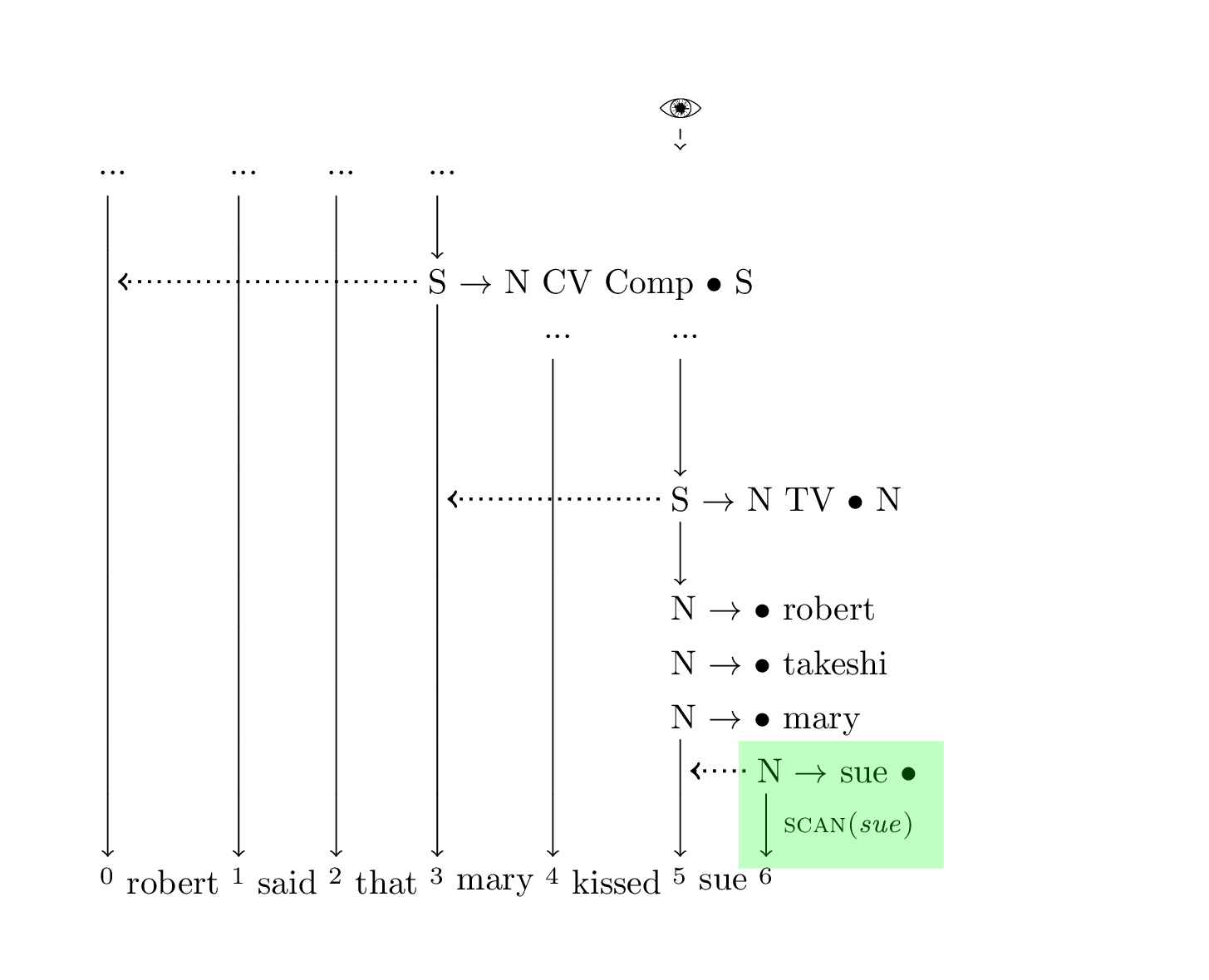```chart := [
(0, N -> • mary)
(0, N -> • sue)
(0, N -> • takeshi)
]
chart := [
(0, N -> robert ••)
(0, S -> N • IV)
(0, S -> N • TV N)
(1, IV -> • fell)
(1, TV -> • kissed)
(1, CV -> • thought)
]
chart := [
(1, CV -> said ••)
]
chart := [
(2, Comp -> that ••)
(0, S -> N CV Comp • S)
(3, N -> • sue)
(3, N -> • robert)
(3, N -> • takeshi)
]
chart := [
(3, N -> mary ••)
(3, S -> N • IV)
(3, S -> N • CV Comp S)
(4, IV -> • fell)
(4, CV -> • said)
(4, CV -> • thought)
]
chart := [
(4, TV -> kissed ••)
(3, S -> N TV • N)
(5, N -> • robert)
(5, N -> • takeshi)
(5, N -> • mary)
]
chart := [ // 👀
(5, N -> sue •)
]
``````chart := [
(0, N -> • mary)
(0, N -> • sue)
(0, N -> • takeshi)
]
chart := [
(0, N -> robert ••)
(0, S -> N • IV)
(0, S -> N • TV N)
(1, IV -> • fell)
(1, TV -> • kissed)
(1, CV -> • thought)
]
chart := [
(1, CV -> said ••)
]
chart := [
(2, Comp -> that ••)
(0, S -> N CV Comp • S)
(3, N -> • sue)
(3, N -> • robert)
(3, N -> • takeshi)
]
chart := [
(3, N -> mary ••)
(3, S -> N • IV)
(3, S -> N • CV Comp S)
(4, IV -> • fell)
(4, CV -> • said)
(4, CV -> • thought)
]
chart := [
(4, TV -> kissed ••)
(5, N -> • robert)
(5, N -> • takeshi)
(5, N -> • mary)
]
chart := [ // 👀
(5, N -> sue ••)
(3, S -> N TV N •)
]
```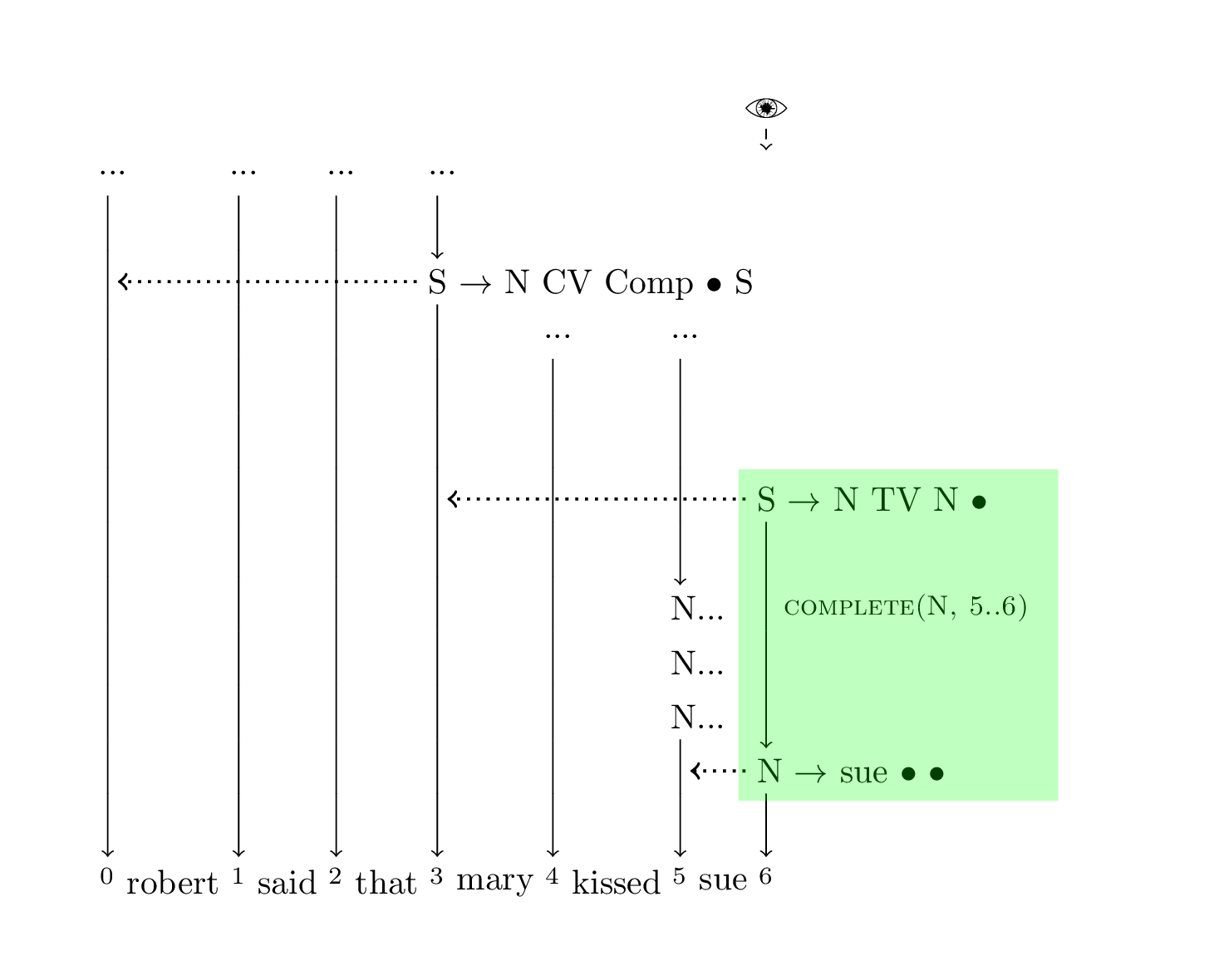```chart := [
(0, N -> • mary)
(0, N -> • sue)
(0, N -> • takeshi)
]
chart := [
(0, N -> robert ••)
(0, S -> N • IV)
(0, S -> N • TV N)
(1, IV -> • fell)
(1, TV -> • kissed)
(1, CV -> • thought)
]
chart := [
(1, CV -> said ••)
]
chart := [
(2, Comp -> that ••)
(3, N -> • sue)
(3, N -> • robert)
(3, N -> • takeshi)
]
chart := [
(3, N -> mary ••)
(3, S -> N • IV)
(3, S -> N • CV Comp S)
(4, IV -> • fell)
(4, CV -> • said)
(4, CV -> • thought)
]
chart := [
(4, TV -> kissed ••)
(5, N -> • robert)
(5, N -> • takeshi)
(5, N -> • mary)
]
chart := [ // 👀
(5, N -> sue ••)
(3, S -> N TV N ••)
(0, S -> N CV Comp S •)
]
```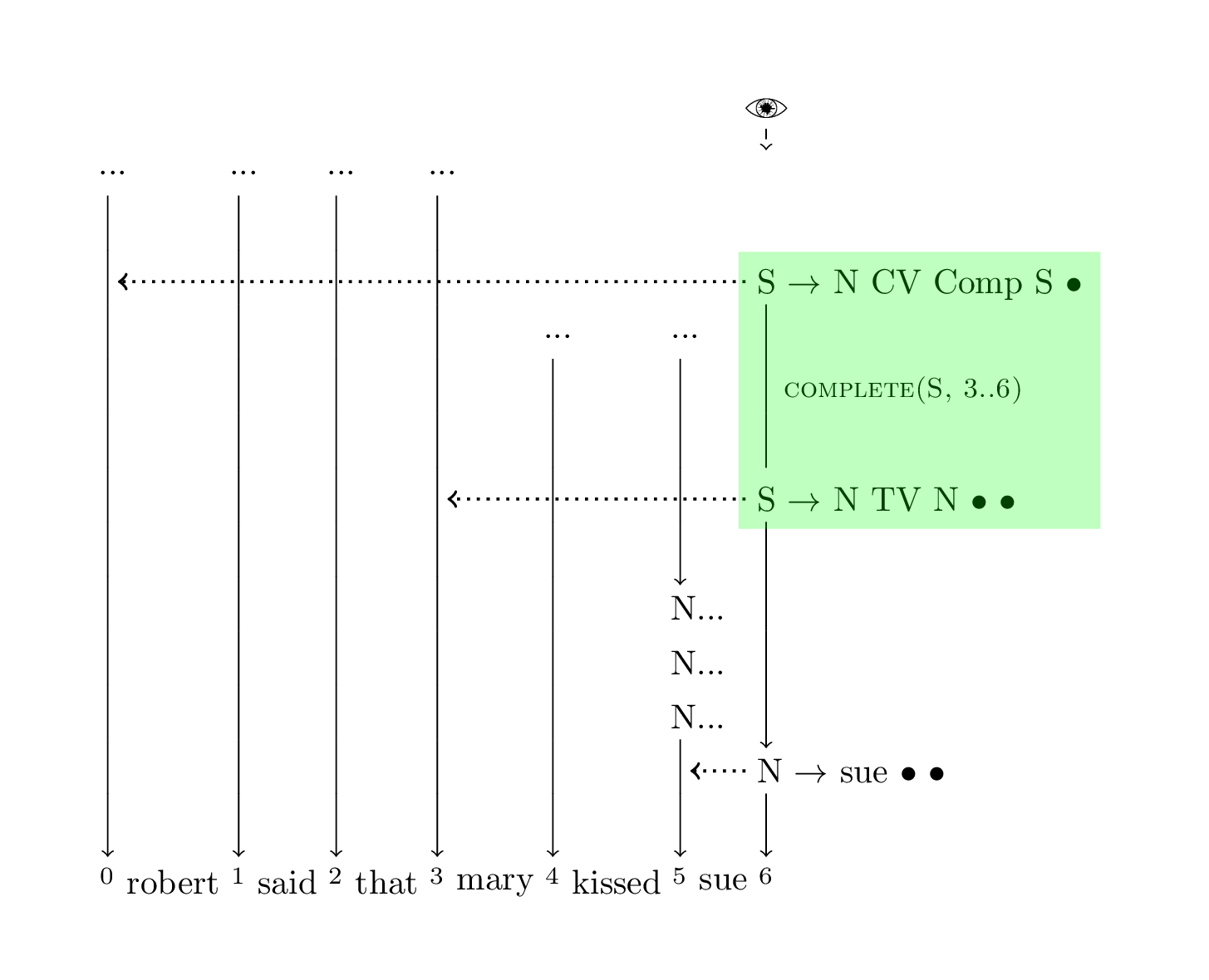```chart := [
(0, N -> • mary)
(0, N -> • sue)
(0, N -> • takeshi)
]
chart := [
(0, N -> robert ••)
(0, S -> N • IV)
(0, S -> N • TV N)
(1, IV -> • fell)
(1, TV -> • kissed)
(1, CV -> • thought)
]
chart := [
(1, CV -> said ••)
]
chart := [
(2, Comp -> that ••)
(3, N -> • sue)
(3, N -> • robert)
(3, N -> • takeshi)
]
chart := [
(3, N -> mary ••)
(3, S -> N • IV)
(3, S -> N • CV Comp S)
(4, IV -> • fell)
(4, CV -> • said)
(4, CV -> • thought)
]
chart := [
(4, TV -> kissed ••)
(5, N -> • robert)
(5, N -> • takeshi)
(5, N -> • mary)
]
chart := [ // 👀
(5, N -> sue ••)
(3, S -> N TV N ••)
(0, S -> N CV Comp S ••)
]
```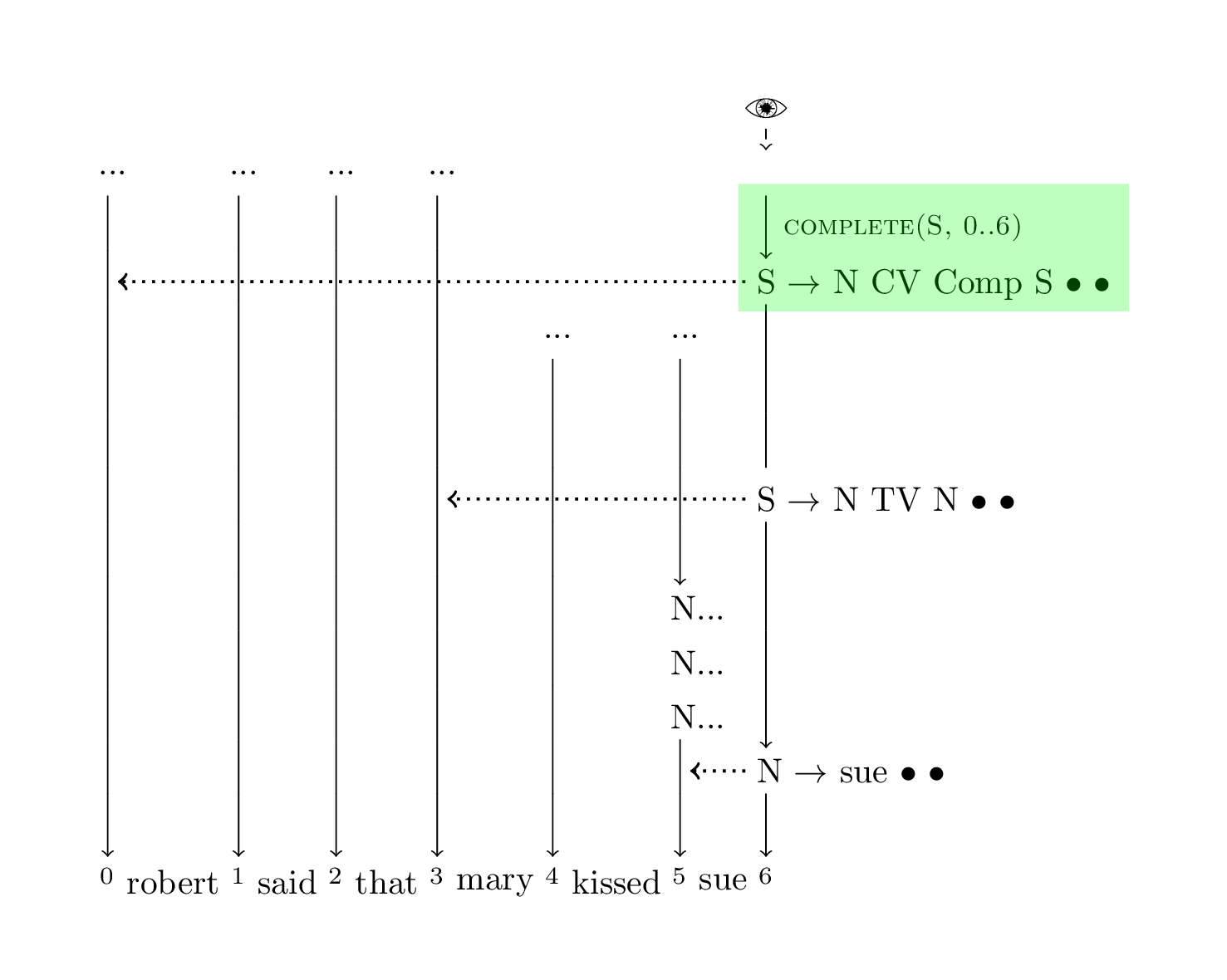```chart := [
(0, N -> • mary)
(0, N -> • sue)
(0, N -> • takeshi)
]
chart := [
(0, N -> robert ••)
(0, S -> N • IV)
(0, S -> N • TV N)
(1, IV -> • fell)
(1, TV -> • kissed)
(1, CV -> • thought)
]
chart := [
(1, CV -> said ••)
]
chart := [
(2, Comp -> that ••)
(3, N -> • sue)
(3, N -> • robert)
(3, N -> • takeshi)
]
chart := [
(3, N -> mary ••)
(3, S -> N • IV)
(3, S -> N • CV Comp S)
(4, IV -> • fell)
(4, CV -> • said)
(4, CV -> • thought)
]
chart := [
(4, TV -> kissed ••)
(5, N -> • robert)
(5, N -> • takeshi)
(5, N -> • mary)
]
chart := [ // 👀
(5, N -> sue ••)
(3, S -> N TV N ••)
(0, S -> N CV Comp S ••)
]
```1

For the curious, if you'll indulge me in making another hasty outlining error, I think the book would look like this:

• Why use symbolic parsing, motivating examples, etc. (expanded version of first part of Part 1)
• LL(k) grammars
• BNF, rewriting, etc.
• Some simple parser like LL
• Show shortcomings of simple parser (null terminals, S -> S S, etc.)
• Context-Free Grammars
• Introduce Earley algorithm
• Earley chart-parsing
• Naive Earley forest
• Show shortcoming of naive Earley forest (S -> S S again)
• Corrected Earley forest (span-based algorithm)
• Show off finished parses, show shortcomings (agreement etc.)
• Features
• Motivating example of features, like case and number agreement
• Introduce features and unification with simple example
• Implement a unification system separate from the parser
• Demo a grammar with features and explain how it will work
• Integrate the unification system into the parser (tree pruning step from `treebender`)
• Possible chapter: type lattice
• `treebender` doesn't actually implement the type lattice, so maybe not, it's not strictly necessary IMO
• Conclusion, talk about production systems (ERG), neural symbolic computing, etc.

One day I'd like to write this.